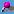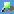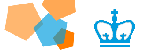Machine Learning 4771 - - Tutorials**Internal**HomeHandoutsNewsStaffSolutionsTutorials **External**ML PeopleCourse related notes Regression by linear combination of basis functions [ps] [pdf] The perceptron [ps] [pdf] Document classification with the multinomial model [ps] [pdf] Sampling from a Gaussian [ps] [pdf] Slides on exponential family distributions [ps] [pdf] Writing in LaTeX A sample document together with its source and a figure [ps] [pdf] [tex] [figure ps]. (In a UNIX/Linux environment use `elatex` to compile, `xdvi` to view and `dvips` to convert to PostScript.) "The not so Short Introduction to LaTeX 2e" by Tobias Oetiker [pdf] "The Comprehensive LaTeX symbol list" by Scott Pakin [ps] [pdf] Further admonishments on writing readable LaTeX documents can be found here. Linear Algebra Review A quick reference for basic operations on vectors and matrices, with the corresponding MATLAB commands indicated [ps] [pdf] Support Vector Machines Tutorial Support vector machines are not in either Bishop's textbook or Jordan's textbook. SVMs are a newer research topic that only really emerged in the later 1990's. To find out more about SVMs, read the following popular tutorial by Chris Burges: A Tutorial on Support Vector Machines for Pattern Recognition [ps] Bayesian Gaussian Tutorial If you want details on integrating all Gaussian models in Bayesian inference (which is a little too advanced for our purposes), feel free to read up on your own in the following optional tutorial also by Tom Minka: Inferring a Gaussian Distribution [ps] Multinomial Tutorial If you are having trouble with multinomial distributions (for counts and discrete data), take a look at Tom Minka's tutorial: Bayesian Inference, Entropy and the Multinomial Distribution Matlab Tutorial Matlab is one of the best tools for designing machine learning algorithms and many of the class assignments and class projects will be easiest to implement and explore with it. Alternatively, it is possible to use other mathematical software like Mathematica or MathCad although these will be much more awkward. Furthermore, it is possible to use C/C++ or Java as the implementation platform but you will require matrix libraries. To install Matlab go to the CS Department website and click www.cs.columbia.edu ->computing ->Software ->Matlab If that doesn't work, Matlab is available to the Columbia community through AcIS. You just connect (i.e. using ssh) to an AcIS CUNIX machine like: ssh -lyourusername cunix.cc.columbia.edu And then run 'matlab' (which lives in /opt/local/bin/matlab). See the following for more details (Windows or Unix): Matlab Software through AcIS The Columbia University Computer Science department also has Matlab available on various Unix machines (in /usr/local/bin/matlab). Matlab Tutorials: Mathworks Matlab documentation MathWorks Matlab Machine Learning Tutorials UNH Matlab Tutorial US Navy Matlab Tutorial MTU Introduction to Matlab A Matlab tutorial on least squares to help with regression. Example code (fits polynomial regression to x,y data): polyreg.m Example code for EM for mixtures of Gaussians (needs the following 4 .m files after): mixmodel.m Example code (generates gaussian samples from a Gaussian mean and covariance matrix): genData.m Example code (plots a 2D Gaussian ellipse contour): plotGauss.m Example code (plots several Gaussians using the above function): plotClust.m Example code (randomly initializes and plots M Gaussians): randInit.m Example code (renders a vector point of gray values as an image, type 'help imageData'): imageData.m Broken code (plays rock-paper-scissors with learning, you add the learning): rockpaper.m Example code (generates samples from a network): sampleNet.m Example code (computes the likelihood of a network): likeNet.m Broken code (learns network from samples, you add the learning): learnNet.m